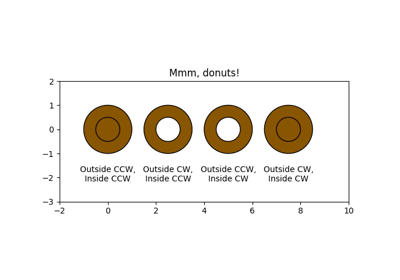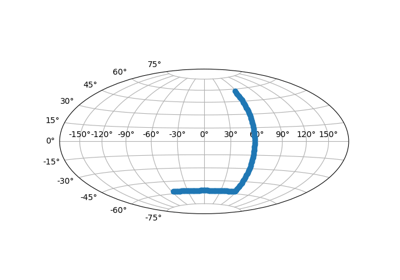/Matplotlib 3.0

# matplotlib.axes.Axes.set_xlim

`Axes.set_xlim(left=None, right=None, emit=True, auto=False, *, xmin=None, xmax=None)`

Set the data limits for the x-axis

Parameters: `left : scalar, optional` The left xlim (default: None, which leaves the left limit unchanged). The left and right xlims may be passed as the tuple (`left`, `right`) as the first positional argument (or as the `left` keyword argument). `right : scalar, optional` The right xlim (default: None, which leaves the right limit unchanged). `emit : bool, optional` Whether to notify observers of limit change (default: True). `auto : bool or None, optional` Whether to turn on autoscaling of the x-axis. True turns on, False turns off (default action), None leaves unchanged. `xmin, xmax : scalar, optional` These arguments are deprecated and will be removed in a future version. They are equivalent to left and right respectively, and it is an error to pass both `xmin` and `left` or `xmax` and `right`. `xlimits : tuple` Returns the new x-axis limits as (`left`, `right`).

#### Notes

The `left` value may be greater than the `right` value, in which case the x-axis values will decrease from left to right.

#### Examples

```>>> set_xlim(left, right)
>>> set_xlim((left, right))
>>> left, right = set_xlim(left, right)
```

One limit may be left unchanged.

```>>> set_xlim(right=right_lim)
```

Limits may be passed in reverse order to flip the direction of the x-axis. For example, suppose `x` represents the number of years before present. The x-axis limits might be set like the following so 5000 years ago is on the left of the plot and the present is on the right.

```>>> set_xlim(5000, 0)
```

## Examples using `matplotlib.axes.Axes.set_xlim`Mmh Donuts!!!Custom projection